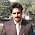# Extract text from alphanumeric value using Excel Formula

Q : Mark asked if we can extract text from alphanumeric value using excel formula.

Solution : Suppose alphanumeric value is placed in cell A2.

Enter the following formula in cell B2.

How it works ?

The Microsoft Excel SUBSTITUTE function replaces a set of characters with another.

Trick : The above formula replaces 1 through 9 with blank value. Hence, all the numeric values are rubbed out from the alphanumeric string.Share
Related PostsDeepanshu founded ListenData with a simple objective - Make analytics easy to understand and follow. He has over 10 years of experience in data science. During his tenure, he has worked with global clients in various domains like Banking, Insurance, Private Equity, Telecom and Human Resource.

3 Responses to "Extract text from alphanumeric value using Excel Formula"
1.Yo need to update the formula as it does not remove number zero from text

=SUBSTITUTE( SUBSTITUTE( SUBSTITUTE( SUBSTITUTE(SUBSTITUTE( SUBSTITUTE( SUBSTITUTE( SUBSTITUTE( SUBSTITUTE(+SUBSTITUTE(A2,"0",""),"1",""),"2",""), "3",""),"4",""),"5",""), "6",""),"7",""),"8",""),"9","")

2.3.Thanks a lot

Next → ← Prev
Love this post? Support Us!